## Calculate Win Loss Tie

Suppose you got a task to calculate the win, loss, and tie totals; what would you do? If you are new to Ms Excel and don’t have enough experience with it, then you might do this task manually but let me add that doing this task manually would take a lot of time you will not be able to complete the task at a specific time and you will end up exhausted.

So for calculating a lot of win, loss, and tie totals in a matter of seconds, all you need to do is to read this article thoroughly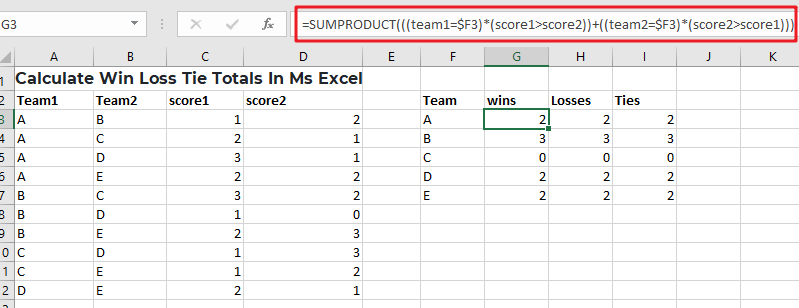So without any further ado, let’s dive into it.

## General Formula

Use the method below to compute win-loss tie totals.

`=SUMPRODUCT(((team1=\$F3)*(score1>score2))+((team2=\$F3)*(score2>score1)))`

## Syntax Explanation

• Parenthesis (): This symbol is used to organize the elements.
• Plus operator (+): This symbol aids in the addition of values.
• SUMPRODUCT: In Excel, this function multiplies the relevant arrays or ranges and returns the total of the products. More information on the SUMPRODUCT Function may be found here.

## Summary

A formula based on the SUMPRODUCT function may be used to determine a team’s win, loss, and tie totals using game data that contains a score for both sides. Type the following formula:

`=SUMPRODUCT(((team1=\$F3)*(score1>score2))+((team2=\$F3)*(score2>score1)))`Based on the data presented, this formula gives total wins for the “A”  team and set the following are the named ranges: team1 (A3:A12), team2 (B3:B12), score1 (C3:C12), and score2 (D3:D12).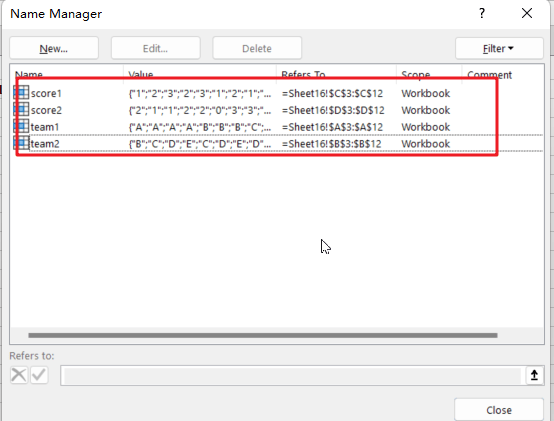### Let’s See How This Formula Works:

In this example, the purpose is to compute total wins, losses, and ties for each team mentioned in column F. The fact that a team can appear in either column A or B complicates matters significantly; therefore, we must account for this when computing wins and losses.

You may consider utilizing the COUNTIF or COUNTIFS function to solve this problem. However, these methods are limited to dealing with established ranges for criteria. Instead, the example formula uses the SUMPRODUCT function to sum the result of a Boolean logic-based array expression. When a team occurs in column A, we use the following equation within SUMPRODUCT on the left:

=((team1=\$F3)*(score1>score2))

This expression comprises two expressions connected by multiplication (*) to construct AND logic.

=(team1=\$F3) / It will verify that if the team is “A”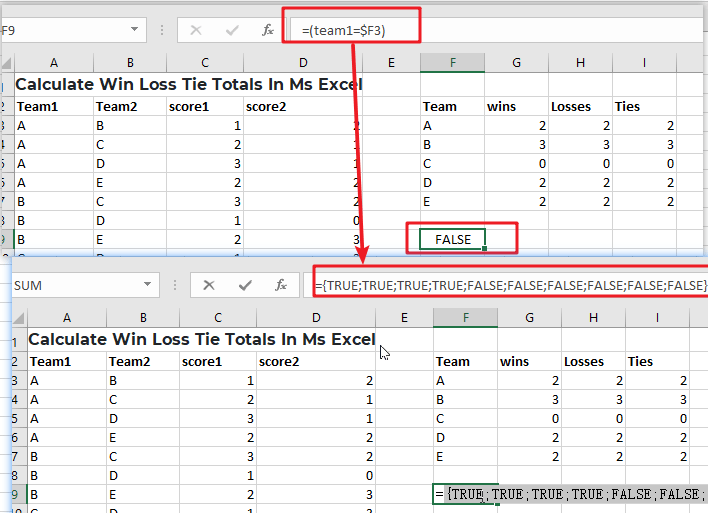The equation on the right determines whether or not the score1 is larger than the score2:

=(score1>score2) / ensure that score1 is larger than the score2.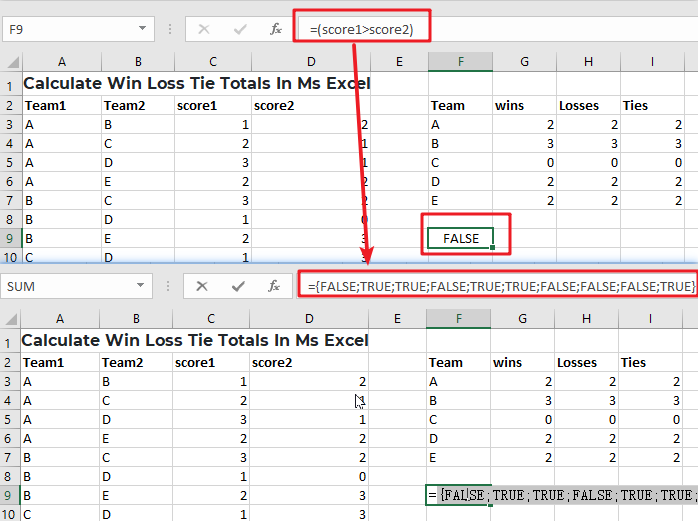Because both expressions utilize multiple-valued ranges, they both yield arrays with numerous outcomes. When we rewrite the formula with the arrays returned.

Multiplication (*) is a math operation that, like AND in Boolean algebra, converts TRUE and FALSE values into 1s and 0s. When both of the related values are TRUE, the outcome is 1. In all other cases, the answer is 0. The end result is a single array that looks like the following: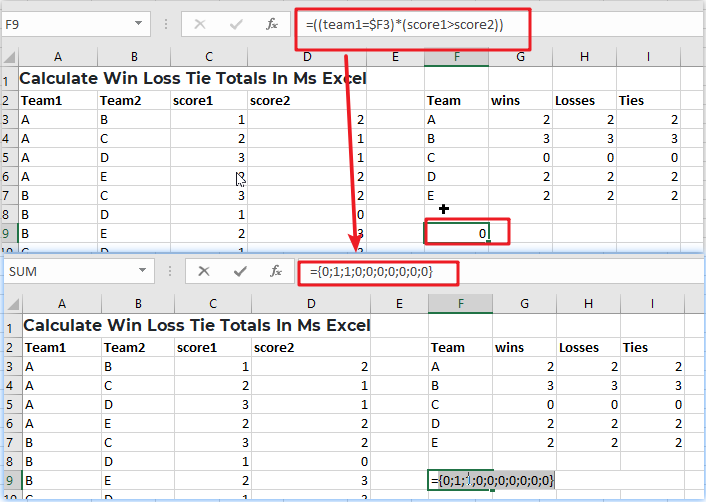We have identical reasoning on the right side that checks for Team A wins when A is Team 2:

=((team2=\$F3)*(score2>score1)))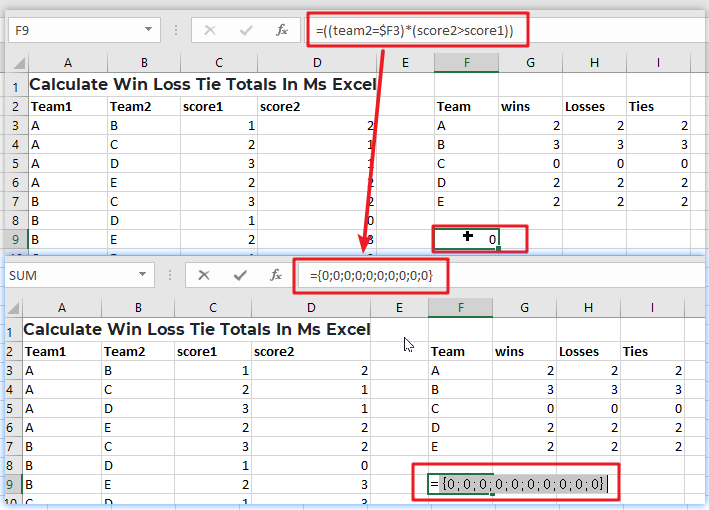Because “A” does not appear as Team 2 in any game, all results are 0.

After evaluating both the left and right sides, we can rewrite the original formula as follows:

`=SUMPRODUCT(({0;1;1;0;0;0;0;0;0;0})+({0;0;0;0;0;0;0;0;0;0}))`

Notice that the arithmetic operator between the two arrays is addition (+), which corresponds to OR logic at this point. We do this because A wins as either Team 1 or Team 2. The result is a single array within SUMPRODUCT:

`=SUMPRODUCT(({0;1;1;0;0;0;0;0;0;0})`

With only one array to process, SUMPRODUCT adds the array’s components and provides a single result, 2.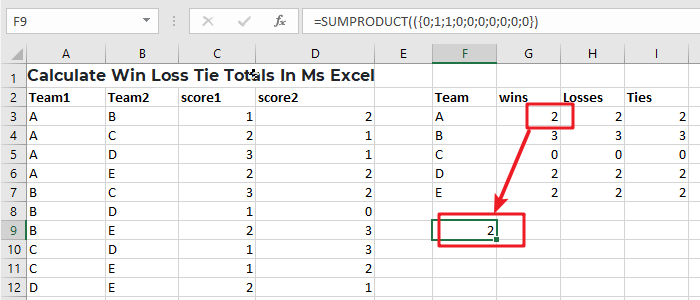### Related Functions

• Excel SUMPRODUCT function
The Excel SUMPRODUCT function multiplies corresponding components in the given one or more arrays or ranges, and returns the sum of those products.The syntax of the SUMPRODUCT function is as below:= SUMPRODUCT (array1,[array2],…)…
• Excel COUNTIF function
The Excel COUNTIF function will count the number of cells in a range that meet a given criteria. This function can be used to count the different kinds of cells with number, date, text values, blank, non-blanks, or containing specific characters.etc.= COUNTIF (range, criteria)…
• Excel COUNTIFS function
The Excel COUNTIFS function returns the count of cells in a range that meet one or more criteria. The syntax of the COUNTIFS function is as below:= COUNTIFS(criteria_range1, criteria1, [criteria_range2, criteria2]…)…

## Average Of Numbers With Multiple Criteria In Excel

Have you ever come across a task to calculate the average of the numbers with respect to multiple criteria? Are you tired of doing this cumbersome task manually? Are you willing to do this task smartly in just a matter of seconds? Then congratulations because you have just landed on the right article.

In this article, you will get to know the easiest way to calculate the average of the numbers with respect to multiple criteria in no time.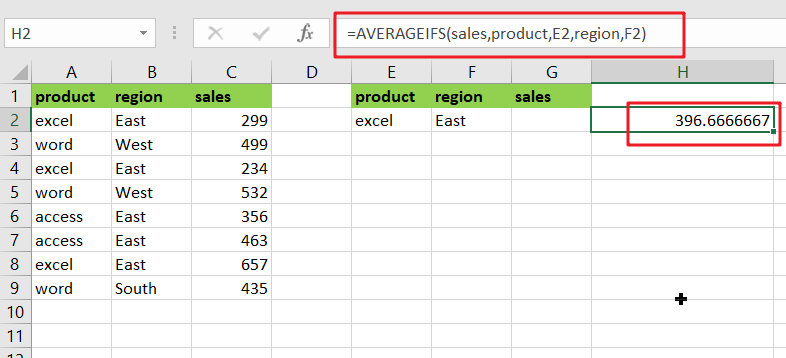## General Formula

`=AVERAGEIFS(values,range1,criteria1,range2,criteria2)`

### Explanations for Syntax:

Before learning how to use the above formula, let’s first understand the role of each syntax in quickly calculating the average of the numbers concerning multiple criteria.

• `AVERAGEIFS`: In Excel, this function returns the average of a set of input values that meet multiple criteria. Learn more about the AVERAGEIFS Function.
• `Comma symbol` (,): It acts as a separator that helps to separate a list of values.
• `Parenthesis` (): The main function of this symbol is to group the elements.
• `Range`: It represents the input value from your worksheet.
• `Criteria`: The specific criteria or values used to calculate the average.

## Explanation

You can use the AVERAGEIFS function to average numbers based on multiple criteria. In the example, the formula in H2  is as follows:

`=AVERAGEIFS(sales,product,E2,region,F2)`Where “sales” (C2:C9), “product” (A2:A9), and “sales” (C2:C9) are named ranges.

The AVERAGEIFS function, like the COUNTIFS and SUMIFS functions, is intended to handle multiple criteria that are entered in [range, criteria] pairs. AVERAGEIFS’s behavior is fully automatic as long as this information is provided correctly.

In the example, we have product values in column E and region values in column F. We use these values directly by using cell references as criteria.

The first argument holds the range of values to average:sales range

To limit the calculation by product, we provide:product, E2

We use the following formula to limit the calculation by region:region, F2

The answer in cell H2 is 396:

Now, let’s go to the 2nd example in which we want to average the product of “excel” and the sales greater than 300. Please follow these instructions:

Enter the formula given below into a blank cell:

`=AVERAGEIFS(sales,product,E2,sales,F2)`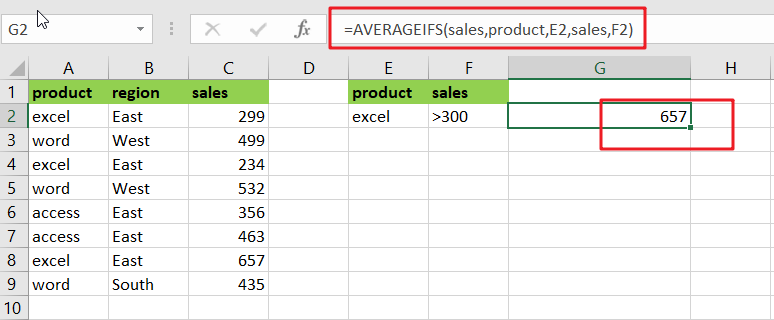(product range is the data that contains the criteria1, sales range is the range which we want to calculate the average, E2 and F2 are the criteria1 and criteria 2), then press the Enter key to get the desired result. Take a look at this screenshot:

Note: If you need more than two criteria, add the criteria ranges and criteria you require as follows:

`=AVERAGEIFS(sales,product,E2,region,F2,sales,G2)`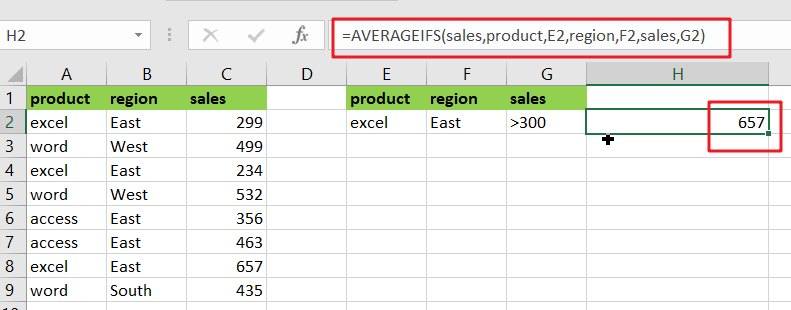Note: the first criteria range is product and E2, the second criteria range is region and F2 and the third criteria is sales and G2 and the range you want to average the values is the criteria, sales range.

For Better understanding, we are adding up the 3rd example as follows:

• Consider the example below to calculate the average of numbers based on multiple criteria.
• The image below will show the input values for Columns B, C, and D.
• Next, enter the given formula in the formula bar section.
• Finally, we’ll see the result in cell H3.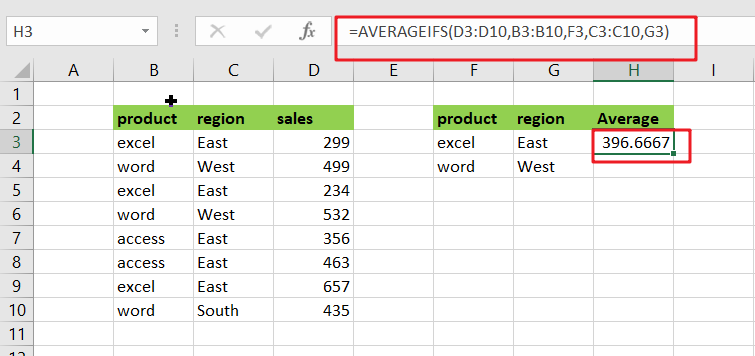## Summary

This article explains how to use the formulas in Excel to calculate the average of numbers with multiple criteria. I hope you found this article interesting. If you have any ideas, please share them with us.

### Related Functions

• Excel AVERAGEIFS function
The Excel AVERAGEAIFS function returns the average of all numbers in a range of cells that meet multiple criteria.The syntax of the AVERAGEIFS function is as below:= AVERAGEIFS (average_range, criteria_range1, criteria1, [criteria_range2, criteria2],…)….
• Excel COUNTIFS function
The Excel COUNTIFS function returns the count of cells in a range that meet one or more criteria. The syntax of the COUNTIFS function is as below:= COUNTIFS(criteria_range1, criteria1, [criteria_range2, criteria2]…)…
• Excel SUMIFS Function
The Excel SUMIFS function sum the numbers in the range of cells that meet a single or multiple criteria that you specify. The syntax of the SUMIFS function is as below:=SUMIFS (sum_range, criteria_range1, criteria1, [criteria_range2, criteria2], …)…

## Count Cells that are Case Sensitive

If you are a frequent user of Microsoft Excel, you may have come across situations where you needed to add or count the cells that were case-sensitive. If you have done this task manually, I would like to point out that it is the most inefficient way to do it, particularly when MS Excel has the versatility to do it in a matter of seconds. As a result of attentively reading this article, you will learn about the many adding or counting case-sensitive cells.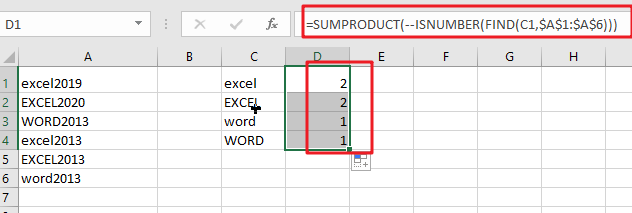Simple generic formula:

```=SUMPRODUCT(--ISNUMBER(FIND(value, range)))
```

## Summary

You may use a formula depending on the ISNUMBER and FIND functions and the SUMPRODUCT function to calculate the number of cells that contain specified text (i.e., hold a substring), taking into consideration upper and lower case. D1 in the example displayed has the following formula, which has been written down:

`=SUMPRODUCT(--ISNUMBER(FIND(C1,\$A\$1:\$A\$6)))`## Let’s See How This Formula Works

In this example, the purpose is to count the number of times each code appears as a substring in a case-sensitive manner, as shown below. When calculating text values, the functions COUNTIF and COUNTIFS are excellent choices. However, since these functions are not case-sensitive, they cannot be utilized to address this issue. To check for substrings, the FIND function should be used in conjunction with the ISNUMBER function, and the SUMPRODUCT function should be used to sum up, the results.

`= FIND(C1,\$A\$1:\$A\$6)`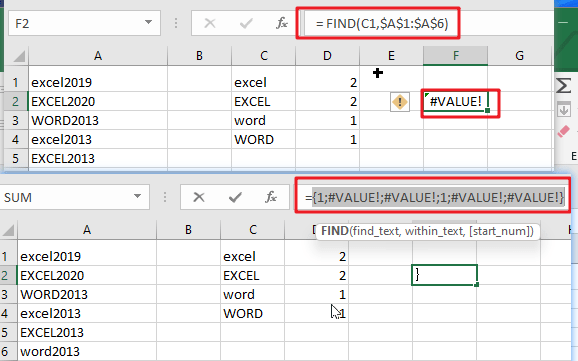The FIND function is case-sensitive and accepts three parameters: find text, within the text, and start num. It is also case-sensitive and takes three arguments. Here is the text we are searching for, and this is the text that we are searching inside. Start num is the number of characters to begin searching in find text. Because start num defaults to 1, we are not supplying a number in this instance. This is necessary since we always want FIND to begin with the first character. When finding the text is discovered inside within text, FIND returns the position of the found text as a number: When finding the text is located within text, FIND returns the position of the found text as a number:

Note: When the find text function fails to yield a result, FIND returns the #VALUE! Error.

`=ISNUMBER(FIND(C1,\$A\$1:\$A\$6))`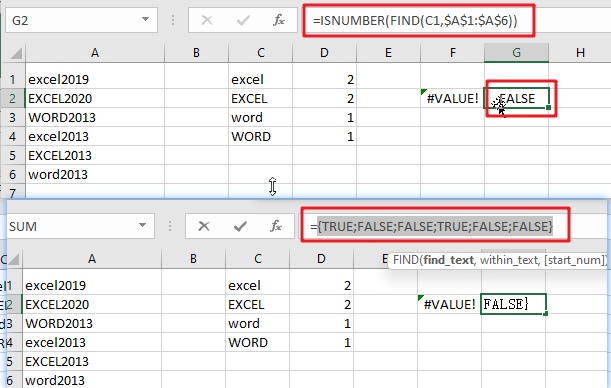This implies that we may be using the ISNUMBER function to transform the output of the FIND function into a TRUE or FALSE value using the ISNUMBER function. A TRUE value will arise from any number, while a FALSE value will result from any error:

As demonstrated in the example, we have four substrings in column C and a variety of codes in columns A1:A6, representing the data for the designated range. Specifically, we want to know how many times each substring in C1:C4 occurs in A1:A6, and we want to know how many times each substring is case-sensitive.

### Related Functions

• Excel SUMPRODUCT function
The Excel SUMPRODUCT function multiplies corresponding components in the given one or more arrays or ranges, and returns the sum of those products.The syntax of the SUMPRODUCT function is as below:= SUMPRODUCT (array1,[array2],…)…
• Excel COUNTIF function
The Excel COUNTIF function will count the number of cells in a range that meet a given criteria. This function can be used to count the different kinds of cells with number, date, text values, blank, non-blanks, or containing specific characters.etc.= COUNTIF (range, criteria)…
• Excel COUNTIFS function
The Excel COUNTIFS function returns the count of cells in a range that meet one or more criteria. The syntax of the COUNTIFS function is as below:= COUNTIFS(criteria_range1, criteria1, [criteria_range2, criteria2]…)…
• Excel ISNUMBER function
The Excel ISNUMBER function returns TRUE if the value in a cell is a numeric value, otherwise it will return FALSE.The syntax of the ISNUMBER function is as below:= ISNUMBER (value)…
• Excel FIND function
The Excel FIND function returns the position of the first text string (sub string) within another text string.The syntax of the FIND function is as below:= FIND(find_text, within_text,[start_num])…

## Check Dates in chronological order

Assume you have a date list that has different date formats, as seen in the accompanying picture. In this instance, Excel’s Sort function will fail to sort them appropriately. However, you may convert all various date formats to a particular format, such as mm/dd/yyy, and then arrange them chronologically. This post will demonstrate how to sort dates in chronological order and how to check if a range of dates are in chronological order.

## Explanation

This is an excellent illustration of how the SUMPRODUCT function may be used to overcome the inability of the COUNTIF or COUNTIFS functions to operate. In this scenario, the purpose is to verify that all dates within a certain range are in chronological order and to display a “YES” value only when dates are in chronological order.

The argument is pretty straightforward, although maybe not apparent. Rather of ensuring that all dates are bigger than the previous one, we determine if any prior date is greater than the next. If we discover even one, the dates will be out of sequence. They are if we discover none (zero).

At its heart, this formula uses the SUMPRODUCT function to determine the number of dates in A1:C1 that are larger than or equal to B1:D1:

`=SUMPRODUCT(--(A1:C1>=B1:D1))`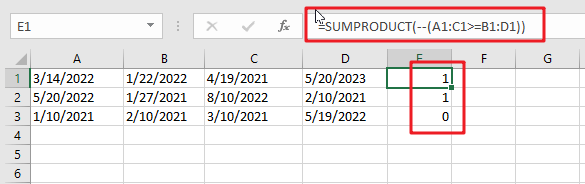Due to the fact that we are employing the larger than or equal to (>=) operator, successive duplicate dates will be disallowed.

This is a two-range operation with 4 dates in each range. As a consequence, an array of TRUE and FALSE values is created as follows: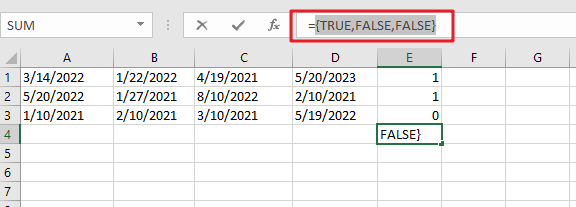You can use the following formula based on the IF function, the SUMPRODUCT function, type:

`=IF(SUMPRODUCT(--(A1:C1>=B1:D1)) =0,”YES”,””)`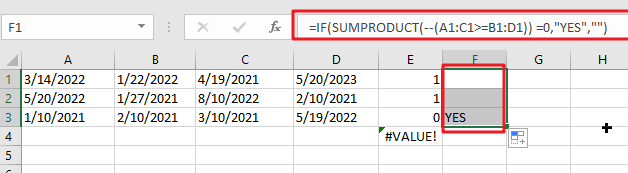In Excel 365, the SORT function offers a convenient alternate solution:

`=IF(SUM(--(A1:D1<>SORT(A1:D1,1,1,1)))=0,"YES","")`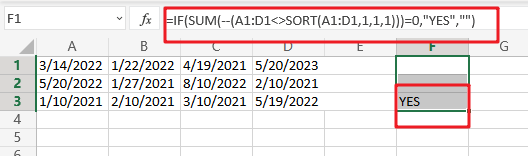The dates in A1:D1 are compared to the same dates following SORT sorting. As with the original algorithm, we are counting any instance when a date is not same (i.e. any date moved by SORT). If there are no dates that vary, a “YES” String Value is returned.

In contrast to the original formula, the SORT version does not check for blank (empty) fields or duplicate dates automatically. The following version has an extra check for blank cells:

`=IF(SUM(((A1:D1<>SORT(A1:D1,1,1,1)))+( A1:D1=""))=0,"YES","")`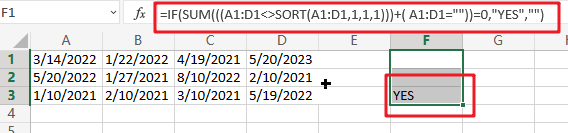In Boolean Algebra, the addition (+) operator behaves similarly to the OR logic. Because the math operation converts TRUE and FALSE values to 1s and 0s automatically, we no longer need the double negative (–).

Note: we use SUM rather than SUMPRODUCT here since Excel 365’s dynamic array support enables SUM to perform array operations natively, without the need for control + shift + enter.

### Related Functions

• Excel SUM function
The Excel SUM function will adds all numbers in a range of cells and returns the sum of these values. You can add individual values, cell references or ranges in excel.The syntax of the SUM function is as below:= SUM(number1,[number2],…)…
• Excel SUMPRODUCT function
The Excel SUMPRODUCT function multiplies corresponding components in the given one or more arrays or ranges, and returns the sum of those products.The syntax of the SUMPRODUCT function is as below:= SUMPRODUCT (array1,[array2],…)…
• Excel COUNTIF function
The Excel COUNTIF function will count the number of cells in a range that meet a given criteria. This function can be used to count the different kinds of cells with number, date, text values, blank, non-blanks, or containing specific characters.etc.= COUNTIF (range, criteria)…
• Excel IF function
The Excel IF function perform a logical test to return one value if the condition is TRUE and return another value if the condition is FALSE. The IF function is a build-in function in Microsoft Excel and it is categorized as a Logical Function.The syntax of the IF function is as below:= IF (condition, [true_value], [false_value])….
• Excel Sort function
The SORT function in Excel sorts the contents of an array or range alphabetically or numerically by columns or rows.The syntax:=SORT(array, [sort index],) …
• Excel COUNTIFS function
The Excel COUNTIFS function returns the count of cells in a range that meet one or more criteria. The syntax of the COUNTIFS function is as below:= COUNTIFS(criteria_range1, criteria1, [criteria_range2, criteria2]…)…

## How to Statistic Pass 4 Out of 5 Rounds in a Competition by Excel Functions

Suppose you are counting the numbers of participants who are the winners in a competition. And the passing line is provided as pass 4 out of 5 rounds of games. You need to know if participant pass the game or not firstly. If you are familiar with the functions in Excel and can use them expertly, you can work out this issue easily. By the way, if you are confused of choosing and applying which function among all Excel functions, this article will help you to solve your problem.

In this article, through explaining the example below, we will introduce you to apply IF and COUNTIF functions together to return win or lose for each participant. You can get the basic knowledge and usage of these two functions in this article.

## EXAMPLE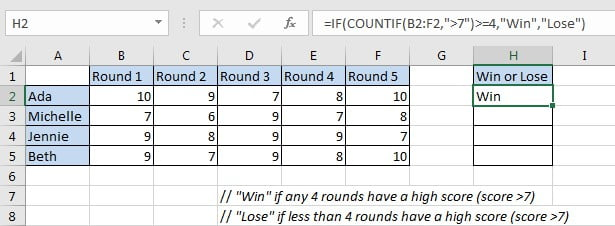In this case, we want to know if participants win or lose the game. “Win” is returned if participant passed four rounds of games with a high score; otherwise “Failed” is returned.

In this article, to approach our goal, we applied IF function with COUNTIF function inside.

## SOLUTION

In this instance, the final output in “Win of Lose” column is “Win” or “False”, the result depends on if participants passed any four rounds of games with high a high score. To output either A or B based on one condition, we can apply IF function here. This function is frequently used in the situations like ‘to return A or B by a given precondition”.

To count number of rounds with a high score, we can select COUNTIF function as it can count with one condition properly.

Besides, COUNTIF has some related functions like COUNT and COUNTIFS. COUNT function returns number of cells which contain a numeric value; COUNTIF is COUNT+IF, it returns number of cells with one condition or criterion; if there are multiple criteria, we can apply COUNTIFS.

## FORMULA with IF & COUNTIF FUNCTION

In H2, we input the formula =IF(COUNTIF(B2:F2,”>7″)>=4,”Win”,”Lose”). After typing, press Enter, verify that “Win” is displayed in H2. As Ada has a high score in round-1, round-2, round-4 and round-5, she passed the game with 4-rounds high scores, so “Win” is returned for her.Drag down handle to fill other cells with the same formula in “Win or Lose” column.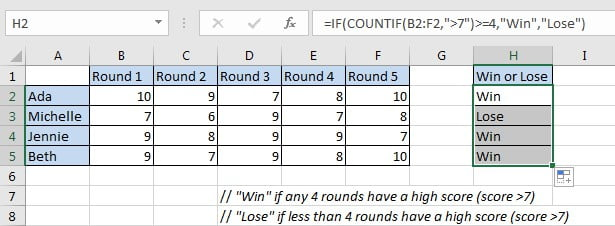Michelle lose the game as she only passes the round-3 and round-5 with a high score.

## FUNCTION INTRODUCTION

In this instance, returning “Win” or “False” depends on the result of whether participants passed four rounds of games with a high score, the final output “Win” of “False” comes from IF function. To determine if participants passed the game with 4 or more high scores, we applied COUNTIF function here.

a. COUNTIF returns the number of cells which contain a number and the number meets one condition or criterion. The condition or criterion is determined by our input in “criteria” argument.

Syntax:

`=COUNTIF(range, criteria)`

Example.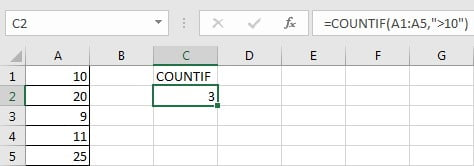b. IF function returns “true value” or “false value” based on the result of provided logical test. It is one of the most popular function in Excel.

Syntax:

`=IF(logical_test,[value_if_true],[value_if_false])`

Example.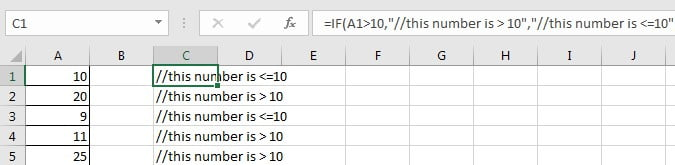## FORMULA EXPLANATION

`=IF(COUNTIF(B2:F2,">7")>=4,"Win","Lose")`

// logical test is “COUNTIF(B2:F2,”>7″)>=4”

// value of true is “Win”

// value of false is “Lose”

c. COUNTIF(B2:F2,”>7″) is used for counting the number of cells from range B2:F2 with the condition number in cell should be “greater than 7”. In this case, this expression is used for counting the rounds number with a high score 8, 9 or 10 for Ada. Expand values in B2:F2, we get below expression:

COUNTIF({10,9,7,8,10},”>7″) // returns 4

d. COUNTIF returned value “4” comes to the logical expression “COUNTIF(B2:F2,”>7″)>=4” inside IF function. In this logical test “4>=4” is true, so the value of true “Win” is returned by this formula finally.

=IF(4>=4,”Win”,”Lose”) // returns “Win”

## EXPAND

If we add two new conditions that round-1 and round-2 must pass with high score, current formula cannot satisfy our demand. Now, the passing line for “Win” is updated to must meet below three conditions:

1. The initial condition “COUNTIF(B2:F2,”>7″)>=4”.
2. Round-1 has a high score “B2>7”.
3. Round-2 has a high score “C2>7”.

Here, we can apply another function AND to concentrate the three conditions in one expression. AND function can return a “TRUE” if all conditions are met.

Update formula to =IF(AND(COUNTIFS(B2:F2,”>7″)>=4,B2>7,C2>7),”Win”,”Lose”).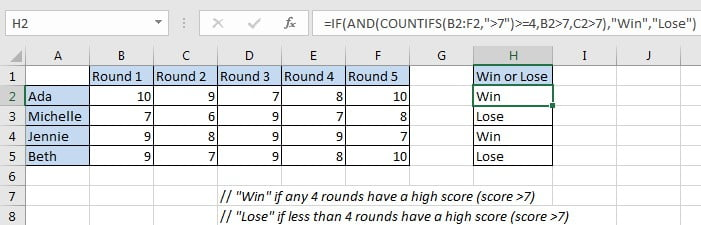### Related Functions

• Excel COUNTIF function
The Excel COUNTIF function will count the number of cells in a range that meet a given criteria. This function can be used to count the different kinds of cells with number, date, text values, blank, non-blanks, or containing specific characters.etc.= COUNTIF (range, criteria)…
• Excel COUNTIFS function
The Excel COUNTIFS function returns the count of cells in a range that meet one or more criteria. The syntax of the COUNTIFS function is as below:= COUNTIFS(criteria_range1, criteria1, [criteria_range2, criteria2]…)…
• Excel IF function
The Excel IF function perform a logical test to return one value if the condition is TRUE and return another value if the condition is FALSE. The IF function is a build-in function in Microsoft Excel and it is categorized as a Logical Function.The syntax of the IF function is as below:= IF (condition, [true_value], [false_value])….
• Excel AND function
The Excel AND function returns TRUE if all of arguments are TRUE, and it returns FALSE if any of arguments are FALSE.The syntax of the AND function is as below:= AND (condition1,[condition2],…)…

## How to Count Not Empty or Blank Cells with COUNTIFS in Excel

This post will guide you how to count not empty cells or categories with COUNTIFS function in Excel 2013/2016 or Excel office 365. You can use the COUNTIFS function to count not blank cells with a given criteria in excel.

## Summary Not Empty Cells with COUNTIFS

Assuming you have a dataset in range A1:C6, and you want to create a formula which can count non-blank cells in column C. Non blank means something has not been enter. And you want column A to be equals a particular item(“excel”) and column B equals to a particular value(“2016”). You may need a specific formula, which will count the number of results for a particular data range on a particular cell. The following formula can be used to build a summary count of non-blank values:

=COUNTIFS(\$A\$1:\$A\$6,”excel”,\$B\$1:\$B\$6,”jan”,\$C\$1:\$C\$6,”<>”)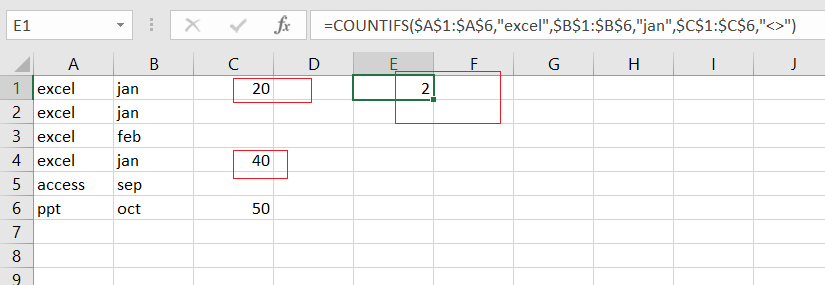As you want a particular value in range A1:A6, and a product value as “excel” is the first criteria. And you also have another date value. And you can notice that there are three conditions. Now what’s great about count is it can count with one or more condition. You just need to put the criteria range, then criteria, and next criteria.

Now for criteria range one and you should have a question in column A. and you need to ask of every cell value in this column , if those cell values are equals to “excel”.

### Related Functions

• Excel SUMPRODUCT function
The Excel SUMPRODUCT function multiplies corresponding components in the given one or more arrays or ranges, and returns the sum of those products.The syntax of the SUMPRODUCT function is as below:= SUMPRODUCT (array1,[array2],…)…
• Excel COUNTIF function
The Excel COUNTIF function will count the number of cells in a range that meet a given criteria. This function can be used to count the different kinds of cells with number, date, text values, blank, non-blanks, or containing specific characters.etc.= COUNTIF (range, criteria)…
• Excel COUNTIFS function
The Excel COUNTIFS function returns the count of cells in a range that meet one or more criteria. The syntax of the COUNTIFS function is as below:= COUNTIFS(criteria_range1, criteria1, [criteria_range2, criteria2]…)…

## How to Create a Summary Count by Month with COUNTIFS in Excel

This post will guide you how to create a summary count by month with COUNTIFS function in Excel 2013/2016 or Excel office 365. You can use the COUNTIF function to count cells with a given criteria in excel. this guide will show you the simple formula used to create a summary count by month with COUNTIFS function and the EDATE function with two criteria in Excel.

## Summary Count by Month with COUNTIFS

Assuming you have a list of data in range B1:B6 that contain date values, and you want to get a total count per month in your summary table(D1:D3) in your worksheet, and you can use a formula based on the COUNTIFS function to generate a total count per month. Like this:

``=COUNTIFS(\$B\$1:\$B\$6,">="&D1, \$B\$1:\$B\$6,"<"&EDATE(D1,1)) ``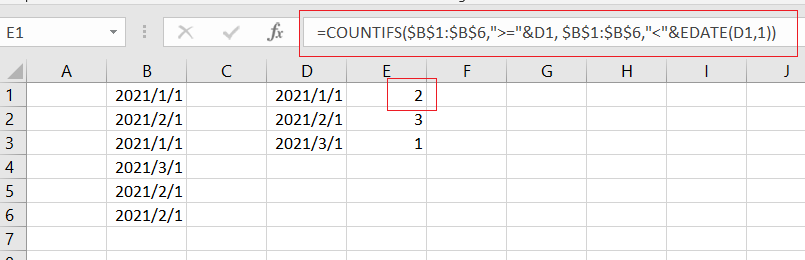Let’s See How This Formula works:

The COUNTIFS function can be used to count the number of cells that meet multiple conditions or criteria. And the Cell D1 is a date for the first of each month in 2021. And if you want to generate a total count per month, and you need to provide criteria that will filter all the date values that appear in each month in range B1:B6.

You still need to build criteria that is a second date can be created with the EDATE function. It means that dates must be greater than or equal to the data in cell D1 or less than the data in D1 plus one month (get it with EDATE function).

### Related Functions

• Excel COUNTIFS function
The Excel COUNTIFS function returns the count of cells in a range that meet one or more criteria. The syntax of the COUNTIFS function is as below:= COUNTIFS(criteria_range1, criteria1, [criteria_range2, criteria2]…)…
• Excel COUNTIF function
The Excel COUNTIF function will count the number of cells in a range that meet a given criteria. This function can be used to count the different kinds of cells with number, date, text values, blank, non-blanks, or containing specific characters.etc.= COUNTIF (range, criteria)…
• Excel EDATE function
TThe Excel EDATE function returns the serial number that represents the date that is a specified number of months before or after a specified date.The syntax of the EDATE function is as below:=EDATE (start_date, months)…

## How to Use COUNTIFS function with Multiple Criteria and OR Logic in Excel

This post will guide you how to Use COUNTIFS function with multiple criteria and OR logic in Excel 2013/2016 or Excel office 365. How to count with multiple criteria and OR logic in Excel.

## COUNTIFS with Multiple Criteria and OR Logic

Normally, when you provide multiple conditions to a COUNTIFS function, and it will check all the conditions and returns rows which matches all the conditions, it means that it runs on AND logic.

If you want to count with multiple conditions and OR logic in your formula, and you can also use the COUNTIFS function to achieve OR logic. Then you still need to use the SUM function and array with multiple condition.

The generic formula is as below:

=SUM(COUNTIFS(range1,{criteria1,criteria2},range2,{criteria3,criteria4}…))

## Example

Assuming that you have a list of data in range B1:B6 in your worksheet, and you wish to count product name which is “excel” or “word”, and you can use the COUNTIFS function with an array constant. The formula is like this:

=SUM(COUNTIFS(B1:B6,{“excel”,”word”}))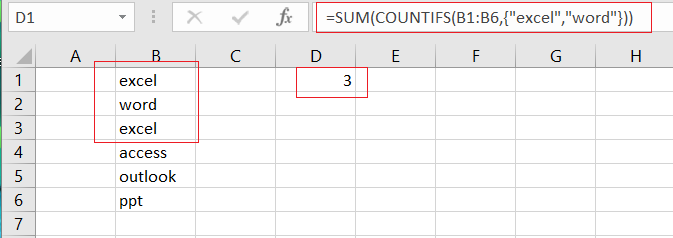Let’s See That How This Formula Works:

=COUNTIFS(B1:B6,{“excel”,”word”})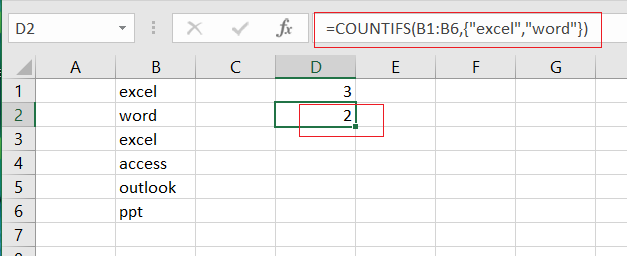The COUNTIFS function can be used to match each item of condition array {“excel”, “word”} and return count of each item in another array list. It means that it will return two values, one is a count for “excel” and another is a count for “word”, the array result is like this:

{2,1}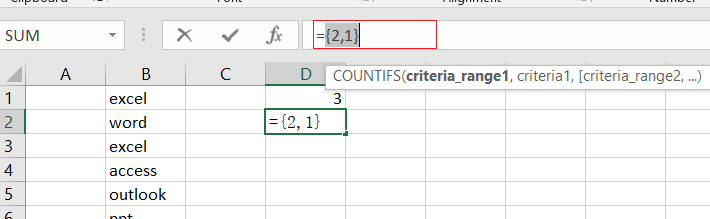Finally, you can use the SUM function to add up all items in an array.

## Adding Two or Multiple Criteria with OR Logic

You can also add two or more criteria to this formula to count multiple criteria using COUNTIFS function with OR logic in Excel. You ‘ll need to add another single column array for one or add another single column array for the other criteria. For example, you wish to count product names that are “excel” or “word”, another criteria is “2016” or “2013”, you can use the below formula:

=SUM(COUNTIFS(B1:B6,{“excel”,”word”},C1:C6,{“2016″,”2013”}))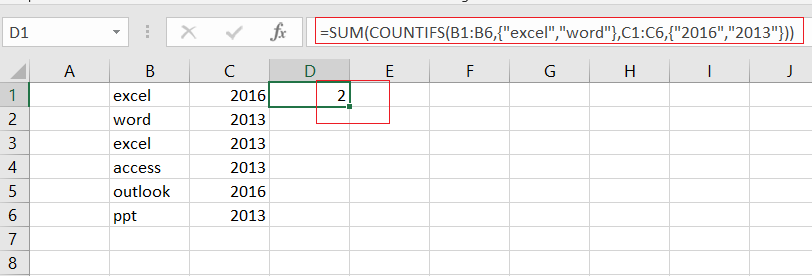### Related Functions

• Excel COUNTIFS function
The Excel COUNTIFS function returns the count of cells in a range that meet one or more criteria. The syntax of the COUNTIFS function is as below:= COUNTIFS(criteria_range1, criteria1, [criteria_range2, criteria2]…)…
• Excel SUM function
The Excel SUM function will adds all numbers in a range of cells and returns the sum of these values. You can add individual values, cell references or ranges in excel.The syntax of the SUM function is as below:= SUM(number1,[number2],…)

## How to Count Number by Range with COUNTIFS Function in Excel

This post will guide you how to Count Number by Range with COUNTIFS function in Excel 2013/2016 or Excel office 365. How do I count the number of values that fall with in a specified range by using the COUNTIFS function in Excel. And you should know that the COUNTIFS function can be used to count the number of cells that meet one or multiple criteria in a given range in Excel. And the post will show you a simple example to count numbers by range with COUNTIFS function.

The Syntax of COUNTIFS function is as below:

=COUNTIFS(range1,criteria1,[range2,criteria2]…)

Where,

Range1 is the data range that will be counted using the criteria1

Criteria1: the condition that determines which cells should be counted

## COUNT NUMBER by Range with COUNTIFS

For Example, you have a data of list in both A2:A6 and B2:B6 and you wish to count the number of sales between the range of 10 to 20, and you can do the following steps:

#1 select Cell D1

#2 enter the following formula based on the COUNTIFS function:

=COUNTIFS(B2:B6,”>=10”,B2:B6,”<=20”)

Then you need to press Enter key to apply this formula. And it will count the cells in range B2:B6 with values between 10 and 20. And the operator “>=” means that “greater than or equal to” and “<=” means that “less than or equal to”.

The formula returns the value 3 in Cell D1. And it means that there are three values between 10 and 20 in your given range B2:B6.

### This formula is the same as in the previous example. And you just only need to change the lowest value is 20, and the highest value is 30. Then this formula will count the cells in range B2:B6 with values between 20 and 30.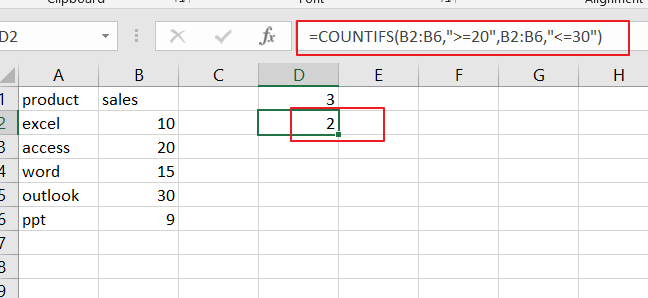### Related Functions

• Excel COUNTIFS function
The Excel COUNTIFS function returns the count of cells in a range that meet one or more criteria. The syntax of the COUNTIFS function is as below:= COUNTIFS(criteria_range1, criteria1, [criteria_range2, criteria2]…)…

## How to Count Specific Items in List in Excel

This post will guide you how to Count Cells that contain a apecific string of text using a formula in Excel 2013/2016 or Excel office 365.How do I create a count of the values that appear in a list or table in Excel. And this post will show you how to use the COUNTIFS function to count items in a list or table in Excel.

The COUNTIFS function extends the COUNTIF function which only allow one criteria. And the COUNTIFS function is similar with the SUMIFS function, which can be used to find the sum of all cells that match two or more criteria.

The Syntax of COUNTIFS function is as below:

=COUNTIFS(range,criteria1,range2,criteria2,…)

Note: range is the first range of cells. Criteria1 is the criteria that be matched against range.

Range2 is the second range of cells that should be matched against criteria.

And if all of criteria is matched in those ranges then a cell is counted.

So you should see that COUNTIFS function works with a pair of range and criteria. And if the all of the given criteria matched, and then the function will return the count of all values in the given range.

Assuming that you have two range of cells A2:A6, and B2:B6. And each value in those two range is criteria. You can specify the below formula to count the number of items in those two ranges.

=COUNTIFS(\$A\$2:\$A\$6,A2,\$B\$2:\$B\$6,B2)

Note: the range A2:A6 is the first range and the criteria is A2. The formula would return a count of every value in range A2:A6.

The range B2:B6 is the second range and the criteria is B2. And this pair would return a count of every value in range B2:B6.

You can see that two different ranges should be used in the above formula.

Type this formula in Cell E2 to get the count of the first row. And then copy down the formula to get the counts of the other rows.

### Related Functions

• Excel COUNTIF function
The Excel COUNTIF function will count the number of cells in a range that meet a given criteria. This function can be used to count the different kinds of cells with number, date, text values, blank, non-blanks, or containing specific characters.etc.= COUNTIF (range, criteria)…
• Excel COUNTIFS function
The Excel COUNTIFS function returns the count of cells in a range that meet one or more criteria. The syntax of the COUNTIFS function is as below:= COUNTIFS(criteria_range1, criteria1, [criteria_range2, criteria2]…)…
• Excel SUMIFS Function
The Excel SUMIFS function sum the numbers in the range of cells that meet a single or multiple criteria that you specify. The syntax of the SUMIFS function is as below:=SUMIFS (sum_range, criteria_range1, criteria1, [criteria_range2, criteria2], …)…

## How to Count the Cells that Match Two Criteria in Excel

This post will guide you how to Count Cells that match two or more criteria in the range of dates using a formula in Excel 2013/2016 or Excel office 365.How do I count on two or multiple criteria or you want to count something in a range of cells only if two or three conditions match in Excel. And you can use the COUNTIFS function to count the number of cells in a range that match a set of multiple criteria in Excel.

The COUNTIFS function extends the COUNTIF function which only allow one criteria. And the COUNTIFS function is similar with the SUMIFS function, which can be used to find the sum of all cells that match two or more criteria.

The Syntax of COUNTIFS function is as below:

=COUNTIFS(range,criteria1,range2,criteria2,…)

Note: range is the first range of cells. Criteria1 is the criteria that be matched against range.

Range2 is the second range of cells that should be matched against criteria2.

And if all of criteria is matched in those ranges then a cell is counted.

## Count the Cells that Match Two Criteria

Assuming that you want to count the number of cells that contain “excel” and price is less than 50. And you have to count on multiple criteria based on COUNTIFS function like this:

=COUNTIFS(A2:A6,”excel”,B2:B6,”<50″)

###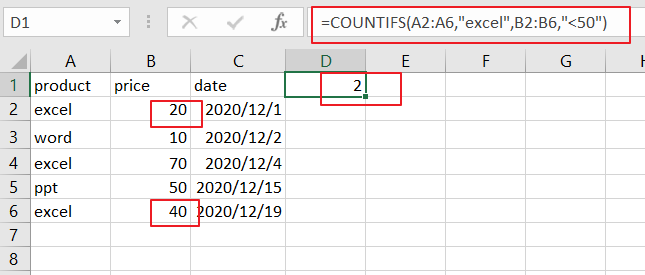You can see that two different ranges should be used in the above formula.

And there is an alternative way of COUNTIFS to count that match two criteria in Excel, you can also use the SUMPRODUCT function to count cells that match multiple criteria. And the equivalent formula like this:

=SUMPRODUCT((A2:A6=”excel”)*(B2:B6<50))

### Related Functions

• Excel SUMPRODUCT function
The Excel SUMPRODUCT function multiplies corresponding components in the given one or more arrays or ranges, and returns the sum of those products. The syntax of the SUMPRODUCT function is as below:= SUMPRODUCT (array1,[array2],…)…
• Excel COUNTIF function
The Excel COUNTIF function will count the number of cells in a range that meet a given criteria. This function can be used to count the different kinds of cells with number, date, text values, blank, non-blanks, or containing specific characters.etc.= COUNTIF (range, criteria)…
• Excel COUNTIFS function
The Excel COUNTIFS function returns the count of cells in a range that meet one or more criteria. The syntax of the COUNTIFS function is as below:= COUNTIFS(criteria_range1, criteria1, [criteria_range2, criteria2]…)…
• Excel SUMIFS Function
The Excel SUMIFS function sum the numbers in the range of cells that meet a single or multiple criteria that you specify. The syntax of the SUMIFS function is as below:=SUMIFS (sum_range, criteria_range1, criteria1, [criteria_range2, criteria2], …)…

## How to Count Cells that Contain Text in Excel

This post will guide you how to count the number of cells that text only within a range of cells using a formula in Excel 2013/2016 or Excel office 365. How do I count the number of cells with text values(not errors, not numbers, not blanks) using a simple formula in Excel. When you analyze a large list of data with texts and numbers, you may also want to know that how many cells that contain text values. The below steps will show you how to do it in a simple way.

## Count Number of Cells that Contain Text

You can use the COUNTIF function to count cells that contain text (not errors, not numbers, not blanks). And you can use the COUNTIF function with a wildcard that matches any number of characters in cells.

The below is a generic formula to count the number of cells containing text:

=COUNTIF(range,*)

Assuming that you have a list of data in range B1:B6 and you want to count the number of cells with text values only. And you can add the the asterisk(*) as a wildcard in the second argument as its criteria and you will get the below formula:

=COUNTIF(B1:B6,”*”)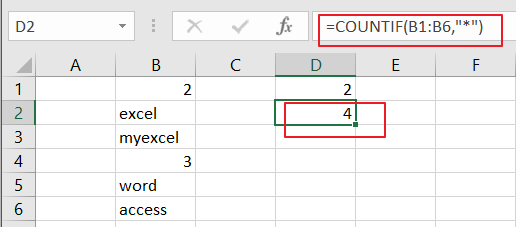LET’S SEE THAT HOW THIS FORMULA WORKS:

The COUNTIF function can be used to count the number of cells that match a single condition or criteria. And in this case, you need to provide a criteria as “*”, which is evaluated as “values that containing any text characters”. Then the total count of all cells in the range B1:B6 that meet the above criteria is returned.

If you want to count cells with text characters in a range of cells, but exclude cells with only a space character, and you can use the COUNTIFS function to supply more specific criteria to achieve the result. Using the below generic formula:

=COUNTIFS(B1:B6,”*”,B1:B6,”<> “)

### Related Functions

• Excel COUNTIF function
The Excel COUNTIF function will count the number of cells in a range that meet a given criteria. This function can be used to count the different kinds of cells with number, date, text values, blank, non-blanks, or containing specific characters. etc. = COUNTIF (range, criteria) …
• Excel COUNTIFS function
The Excel COUNTIFS function returns the count of cells in a range that meet one or more criteria. The syntax of the COUNTIFS function is as below:= COUNTIFS(criteria_range1, criteria1, [criteria_range2, criteria2]…)…

## How to Count Cells Not equal to X or Y in Excel

This post will guide you how to count the number of cells not equal to criteria X or Y in a given range cells using a formula in Excel 2013/2016.You can easily to count cells equal to or not equal to a specific value through COUNTIF function. But if there is an easy way to count cells not equal to either one value or another value in a selected range of cells in Excel.

## Count Cells Not equals to Criteria X or Y

Assuming that you want to count cells that do not contain value “EXCEL”or “word” defined in a selected range(A1:B6).In this case, you can use the COUNTIFS function with multiple criteria to count cells not equal to “EXCEL” or “word”.

Enter the following formula in a blank cell, and press Enter key:

=COUNTIFS(A2:A6,”<>EXCEL”,A2:A6,”<>word”)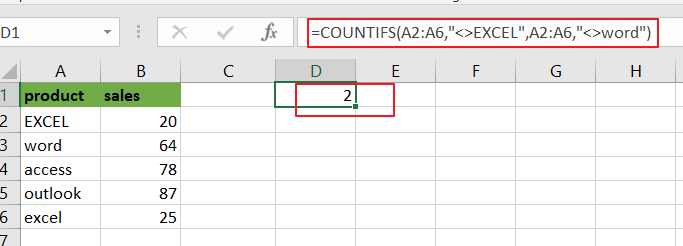Note: A2:A6 is the data range that you want to use. And both“EXCEL” and “word” are the text values that you want to count in range A2:A6. You need to change them as you need.

Now Let’s see how this formula works:

The COUNTIFS function will count the number of cells in a given range that match supplied criteria. All criteria must match while counting. In the above formula, there are two criteria. One criteria is “<>EXCEL”, it means if cell is not equal to “, another is “<>word”, it means that cells should not equal to “word”.

You can also use another array formula to achieve the same result of counting cells not equal to either “EXCEL” or “word” in A2:A6. Just using the following formula based on the SUMPRODUC function:

=SUMPRODUCT((A2:A6<>”EXCEL”)*(A2:A6<>”word”))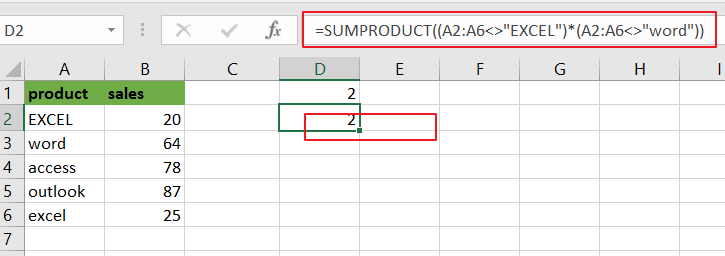### Related Functions

• Excel SUMPRODUCT function
The Excel SUMPRODUCT function multiplies corresponding components in the given one or more arrays or ranges, and returns the sum of those products. The syntax of the SUMPRODUCT function is as below:= SUMPRODUCT (array1,[array2],…)…
• Excel COUNTIFS function
The Excel COUNTIFS function returns the count of cells in a range that meet one or more criteria. The syntax of the COUNTIFS function is as below:= COUNTIFS(criteria_range1, criteria1, [criteria_range2, criteria2]…)…

## How to Count Cells Not Equal to One of Many Things in Excel

This post will guide you how to count the number of cells that are not equal to many things in a given range cells using a formula in Excel 2013/2016. You can easily to count cells not equal to a specific value through COUNTIF function. But if there is an easy way to count cells which are not equal to one of many values defined in a selected range of cells in Microsoft Excel. You can count cells that are not equal to many things with a combined formula based on the SUMPRODUCT function, the ISNA function and the MATCH function.

## Count Cells not equal to one of many Things

Actually, if you just have two or three things that you don’t want to count in your data list, and you can only use one COUNTIFS function like below:

=COUNTIFS(A2:A6,”<>excel”,A2:A6,”<>word”)

The above formula does not scale very well if you have a list of many things that you do not count, since you have to add more range and criteria pair to for each thing that you do not want to count in range. In this case, you can use the MATCH function to find cells in range A2:A6 that are not equal to values in range D1:D2 like this:

=MATCH(A2:A6,D1:D2,0)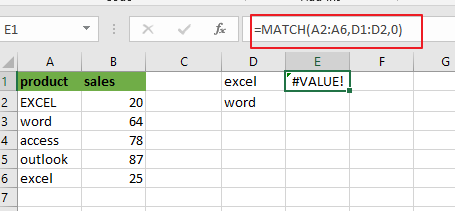Pressing “`Fn`” + “`F9`” or “`F9`” short key to display the array results like this:

={1;2;#N/A;#N/A;1}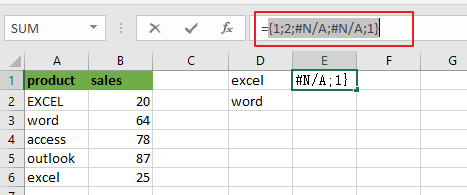A2:A6 is the lookup value and D1:D2 is the lookup array. Since there are more than one lookup value in range D1:D2, so it returns more than one result in an array result like below:

MATCH function returns the position of lookup values in the lookup array, if lookup value is not in lookup array, it will return #N/A errors. Actually, the numbers of “#N/A” is the number of cells that are not equal to things in D1:D2.

Then you can use the ISNA function to convert all values in the above array result to TRUE or FALSE, like below:

=ISNA(MATCH(A2:A6,D1:D2,0))

={FALSE;FALSE;TRUE;TRUE;FALSE}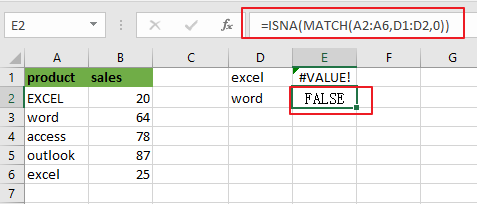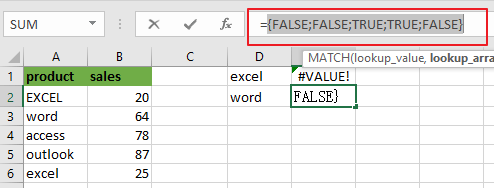Next, you need to use a double negative operator to convert TRUE or FALSE value as 1 or 0 in the above array result. Like below:

=–ISNA(MATCH(A2:A6,D1:D2,0))

={0;0;1;1;0}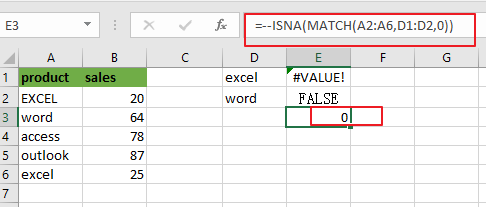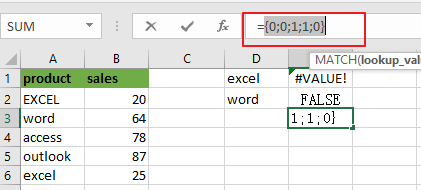Last, passing the above resulting array to the SUMPRODUCT or SUM function looks like below:

=SUMPRODUCT(–ISNA(MATCH(A2:A6,D1:D2,0)))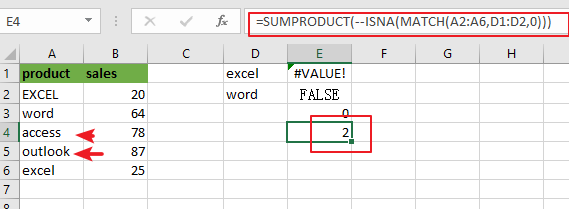Or

{=SUM(–ISNA(MATCH(A2:A6,D1:D2,0)))}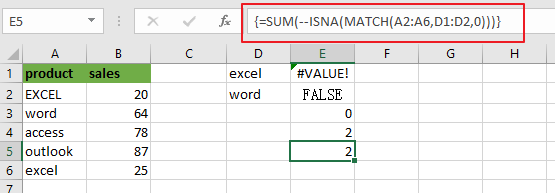Note: if you are using SUM function in the above formula, you need to press “`CTRL + SHIFT + ENTER`” shortcuts to convert the formula as a array formula. And the SUMPRMDUCT function can handle the array result by itself.

You can also use another method to count cells which are not equal to many things with a combined formula that utilizes the COUNTA, SUMPRODUCT, and COUNTIF functions.

The general formula is like this:

=COUNTA(range)-SUMPRODUCT(COUNTIF(range, things))

In this case, use the following formula:

=COUNTA(A2:A6)-SUMPRODUCT(COUNTIF(A2:A6, D1:D2))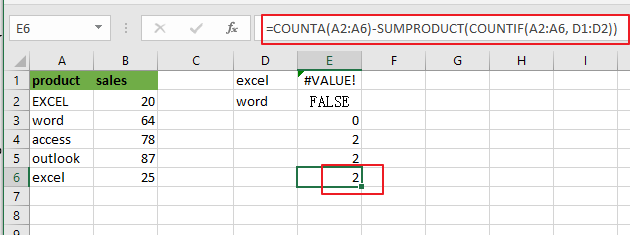Let’s see how this formula works:

The COUNTA function will count all of the cells in the range A2:A6. And the total number of cell in range A2:A6 is 5.

The COUNTIF function will check the range A2:A6 that contain the values in range D1:D2. Then it returns an array result like this:

=COUNTIF(A2:A6, D1:D2)

={2;1}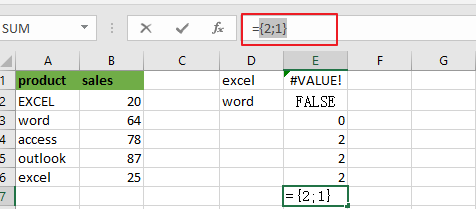The SUMPRODUCT function adds up all numbers in the above resulting array, then the returned number is subtracted from the count of all the values in range A2:A6.

The solution logic is that calculating the count of cells that having specific values and then subtract it from the count of all non-empty cells in range A2:A6.

### Related Functions

• Excel SUMPRODUCT function
The Excel SUMPRODUCT function multiplies corresponding components in the given one or more arrays or ranges, and returns the sum of those products. The syntax of the SUMPRODUCT function is as below:= SUMPRODUCT (array1,[array2],…)…
• Excel COUNTIF function
The Excel COUNTIF function will count the number of cells in a range that meet a given criteria. This function can be used to count the different kinds of cells with number, date, text values, blank, non-blanks, or containing specific characters. etc. = COUNTIF (range, criteria) …
• Excel MATCH  function
The Excel MATCH function search a value in an array and returns the position of that item.The MATCH function is a build-in function in Microsoft Excel and it is categorized as a Lookup and Reference Function.The syntax of the MATCH function is as below:= MATCH  (lookup_value, lookup_array, [match_type])….
• Excel COUNTIFS function
The Excel COUNTIFS function returns the count of cells in a range that meet one or more criteria. The syntax of the COUNTIFS function is as below:= COUNTIFS(criteria_range1, criteria1, [criteria_range2, criteria2]…)…
• Excel SUM function
The Excel SUM function will adds all numbers in a range of cells and returns the sum of these values. You can add individual values, cell references or ranges in excel.The syntax of the SUM function is as below:= SUM(number1,[number2],…)
• Excel ISNA function
The Excel ISNA function used to check if a cell contains the #N/A error, if so, returns TRUE; otherwise, the ISNA function returns FALSE.The syntax of the ISNA function is as below:=ISNA(value)….
• Excel COUNTA function
The Excel COUNTA function counts the number of cells that are not empty in a range. The syntax of the COUNTA function is as below:= COUNTA(value1, [value2],…)…

## How to Count Cells between Two Numbers in Excel

This post will guide you how to count cells between two numbers using a formula in Excel 2013/2016. How do I count times between a given range cells based on a condition in Excel. Is there a easy way to count cells between two given numbers in a selected range of cells in Excel.

## Count Cells between Numbers using COUNTIFS Function

Assume that you have a list of data in range A1:B6, and you want to count cells between two numbers. In this case, and you can use the COUNTIFS function to achieve the result of counting times between two given numbers in Excel. You should know that the COUNTIFS function will count the number of cells that meet one or more criteria. And the below tutorial will help all levels of Excel users in counting cells between two given numbers in your worksheet.

You can use the following formula based on the COUNTIFS function to count cells between 40 and 90.

=COUNTIFS(B2:B6,”>=40″, B2:B6,”<=90″)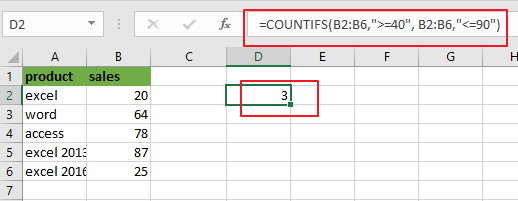From the above screenshot, and you can see that this formula will count sales number in range B2:B6 that are greater than or equal to 40 and less than or equal to 90. To count cells between two numbers, and you need to provide two criteria in COUNTIFS formula. One is for starting number and one is for the ending number.

Let’s see how this formula works:

The COUNTIFS function can be used to count cells that meet one or more criteria in the given range cells.

This formula has two criteria. It counts the cells in range of cells B2:B6 with values between 40 and 90. The operator “>=” means “greater than or equal to”, and “<=” means “less than or equal to”.

The formula will return the value “3”, which indicates to that there are three cells with values between 40 and 90 in your range of cells.

## Count Cells between Numbers using COUNTIF Function

You can also use another function called COUNTIF in an older version of Microsoft Excel to achieve the result of counting cells between two numbers. For the example above you can use the below formula based on the COUNTIF function:

=COUNTIF(B2:B6,”>=40″)-COUNTIF(B2:B6,”>90″)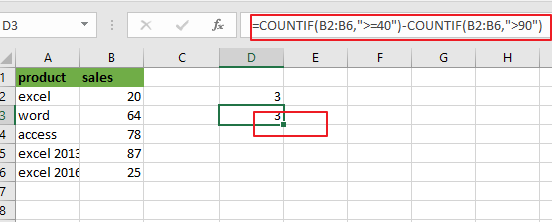Let’s see that how this formula works:

The first COUNTIF function will count the number of cells in a range that are greater than or equal to “40”. And the second COUNTIF function will count the number of cells with values that are greater than “90”. And the second number is subtracted from the first result that returned by the first COUNTIF function. Then you should get the number of cells that contain values between 40 and 90.

The First COUNTIF function:

=COUNTIF(B2:B6,”>=40″)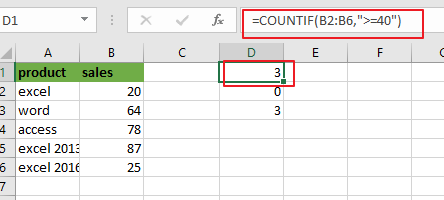The Second COUNTIF function:

= COUNTIF(B2:B6,”>90″)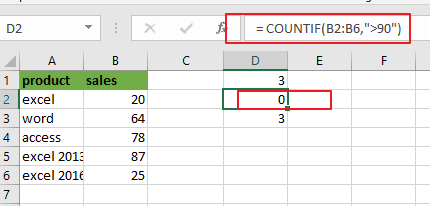### Related Functions

• Excel COUNTIF function
The Excel COUNTIF function will count the number of cells in a range that meet a given criteria. This function can be used to count the different kinds of cells with number, date, text values, blank, non-blanks, or containing specific characters.etc.= COUNTIF (range, criteria)…
• Excel COUNTIFS function
The Excel COUNTIFS function returns the count of cells in a range that meet one or more criteria. The syntax of the COUNTIFS function is as below:= COUNTIFS(criteria_range1, criteria1, [criteria_range2, criteria2]…)…

## How to Count Cells between Two Dates or Times in Excel

This post will guide you how to count cells between two dates using a formula in Excel 2013/2016. How do I count times between a given time or date range based on a condition in Excel. Is there a easy way to count cells between two given dates in a selected range of cells in Excel.

## Count Cells between Dates

Assume that you have a list of data in range A1:B6, and you want to count cells which between 2020/1/1 and 2020/12/31. In the below steps, and you should learn that how can we use COUNTIF function to achieve the result of counting times between two given dates in Excel.

You can use the following formula based on the COUNTIF function:

=COUNTIFS(B2:B6,”>=”&DATE(2020,1,1),B2:B6,”<=”&DATE(2020,12,31))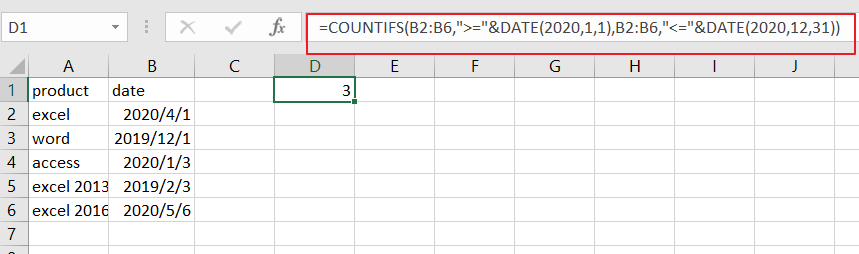Let’s see how this formula works:

The COUNTIFS function can be used to count cells that meet one or more criteria in the given range cells.

From the above screenshot, and you can see that this formula will count sales date in the year 2020, that appear in the range B2:B6. To count cells between two dates, and you need to provide two criteria in COUNTIFS formula. One is for start date and one is for the end date. And the range of cells that you want to compare is B2:B6.

And you still need to construct both starting date and ending date for comparing in the above COUNTIFS formula. You can use the DATE function to do it. Like below:

=DATE(2020,1,1)

=DATE(2020,12,31)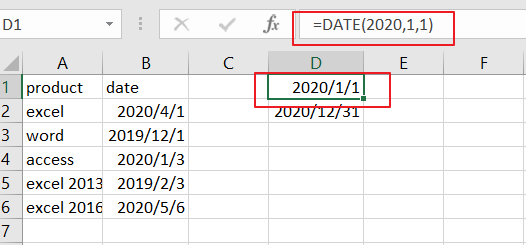You also need to concatenate “greater than or equal to” or “smaller than or equal to” operators with concatenate operator to build those two conditions.

=”>=”&DATE(2020,1,1)

=”<=”&DATE(2020,12,31)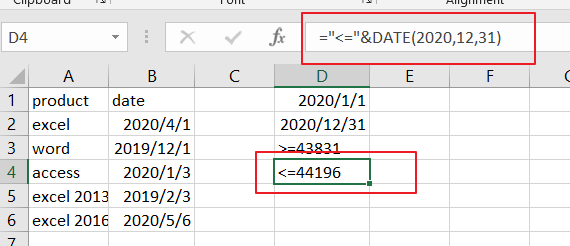Then you can combine these formulas into one like as below:

= B2:B6,”>=”&DATE(2020,1,1),B2:B6,”<=”&DATE(2020,12,31)

This formula can be added into the COUNTIFS formula as its criteria. It performs AND logic because it must be satisfied for both two conditions.

### Related Functions

• Excel COUNTIFS function
The Excel COUNTIFS function returns the count of cells in a range that meet one or more criteria. The syntax of the COUNTIFS function is as below:= COUNTIFS(criteria_range1, criteria1, [criteria_range2, criteria2]…)…
• Excel DATE function
The Excel DATE function returns the serial number for a date.The syntax of the DATE function is as below:= DATE (year, month, day)…

## Count Dates in Given Year/Month/Day in Excel

This post will guide you how to count dates in a give year or month or day with a formula in Excel. How do I count cells based on year, month or day in Excel. How to countif by a specified date (Year or month) in a range of cells in Excel.

## Count Dates in Given Year

Assuming that you have a list of data in range B1:B6 that contain date values, and you want to count those dates by a specified year(2018), how to achieve it. or you want to count the dates by a specified month (5).

You can create a formula based on the SUMPRODUCT function and the YEAR function to count dates by a give year. Just like this:

`=SUMPRODUCT(1*(YEAR(B1:B6)=2018))`

Type this formula into a blank cell and then press Enter key to apply this formula.

###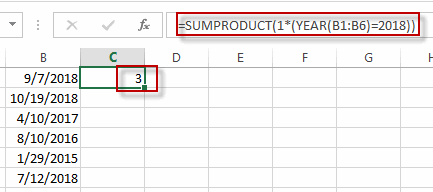The count number is calculated by this formula in Cell C1.

You can also use another excel array formula based on the SUM function , the IF function and the YEAR function to achieve the same result.

`=SUM(IF(YEAR(B1:B6)=2018,1))`

The Year function will convert the data value to a year value only. and the IF function will check if the year value returned by the YEAR function is equal to the given year, if so, return value 1. otherwise, returns nothing. and the sum function will sum the array list that returned by the IF function.

You need to type this formula into a cell, and then press Ctrl + Shift + Enter keys in your keyboard to convert this formula as a array formula.

## Count Dates in a given Month

To count dates in a given month, you can create a formula based on the SUMPRODUCT function and the Month function. For example, you want to count datas in range of cells B1:B6 by a given month value 5. you can write down this formula:

`=SUMPRODUCT(1*(MONTH(B1:B6)=5))`

###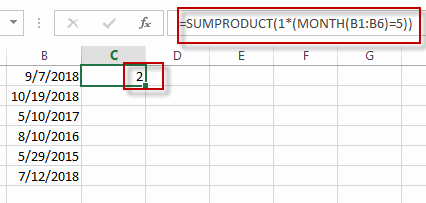Or you can also write down an excel array formula based on the SUM function, the IF function and the MONTH function. Just like this:

`=SUM(IF(MONTH(B1:B6)=5,1))`

## Count dates in a given date(year,month, day)

If you want to count the number of a give date (9/7/2018) in a range of cells (B1:B6) in Excel, You can use a formula based on the COUNTIF function. Just like this:

`=COUNTIF(B1:B6,"9/7/2018")`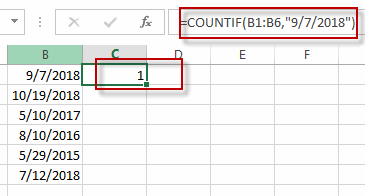## Count Cells between Dates

If you want to count the number of cells that contain dates between two specified dates, You can still use the COUNTIFS function to create a formula to achieve the result.
For example, you want to count the number of cells that fall between two dates(from 1/1/2017 to 10/1/2018), and you can use a fromula based on the countif function. Like this:

```=COUNTIFS(B1:B6,">"&"1/1/2017",B1:B6,"<"&"10/1/2018")
```

### Related Functions

• Excel SUM function
The Excel SUM function will adds all numbers in a range of cells and returns the sum of these values. You can add individual values, cell references or ranges in excel.The syntax of the SUM function is as below:= SUM(number1,[number2],…)…
• Excel IF function
The Excel IF function perform a logical test to return one value if the condition is TRUE and return another value if the condition is FALSE. The IF function is a build-in function in Microsoft Excel and it is categorized as a Logical Function.The syntax of the IF function is as below:= IF (condition, [true_value], [false_value])….
• Excel COUNTIF function
The Excel COUNTIF function will count the number of cells in a range that meet a given criteria. This function can be used to count the different kinds of cells with number, date, text values, blank, non-blanks, or containing specific characters.etc.= COUNTIF (range, criteria)…
• Excel SUMPRODUCT function
The Excel SUMPRODUCT function multiplies corresponding components in the given one or more arrays or ranges, and returns the sum of those products.The syntax of the SUMPRODUCT function is as below:= SUMPRODUCT (array1,[array2],…)…
• Excel YEAR function
The Excel YEAR function returns a four-digit year from a given date value, the year is returned as an integer ranging from 1900 to 9999. The syntax of the YEAR function is as below:=YEAR (serial_number)…
• Excel MONTH function
The Excel MONTH function returns the month of a date represented by a serial number. And the month is an integer number from 1 to 12. The syntax of the MONTH function is as below:=MONTH (serial_numbe…
• Excel COUNTIFS function
The Excel COUNTIFS function returns the count of cells in a range that meet one or more criteria. The syntax of the COUNTIFS function is as below:= COUNTIFS(criteria_range1, criteria1, [criteria_range2, criteria2]…)…

## COUNTIF with Multiple Criteria

This post will guide you how to countif multiple criteria on the same column in excel. How do I countif multiple criteria on the different columns in excel. How to use COUNTIF to count cells that match multiple criteria on the same column or the different columns in Excel.

## COUNTIF with Multiple Criteria in the Same column

Assuming that you have a list of data in column A and you want to count the number of two specified text string (excel and word) in range A1:A5, how to achieve it.

To count the number based on multiple criteria in the same range or column in Excel, you can create a formula based on the SUMPRODUCT function and the COUNTIF function. Just like this:

`=SUMPRODUCT(COUNTIF(A1:A5,{"excel";"word"}))`

Then type this formula into a blank cell and then press Enter key in your keyboard to apply this formula.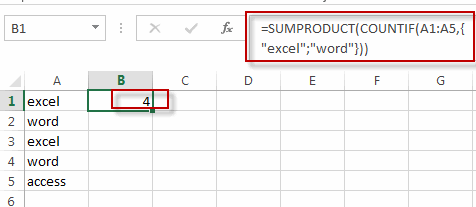You will see that the count number is displayed in that cell.

And if you want to add one more criteria in this formula, for example, you need to count the number of three text strings in the given range, you just add that text string into the formula as below:

`=SUMPRODUCT(COUNTIF(A1:A5,{"excel";"word";"access"}))`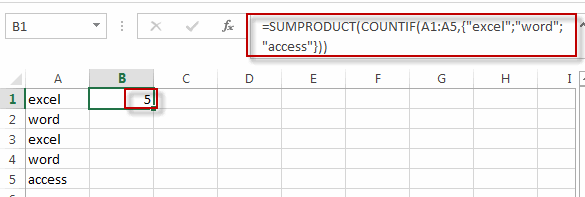Or you can also use another formula based on the SUM function and the COUNTIFS function to achieve the same result. Like this:

`=SUM(COUNTIFS(A1:A5,{"excel","word"}))`

Type this formula into a blank cell, and then press Enter key.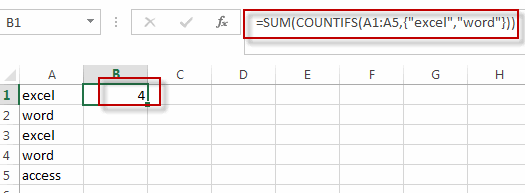## COUNTIF with Multiple Criteria in the Same column

Assuming that you have two lists of data in Range A1:B5, and one is the product name and another is sale values. And you want to count the number of product “excel” and its sale value is greater 30. How to achieve it. You can use the COUNTIFS function to create a formula to achieve the result.

Like this:

`=COUNTIFS(A1:A5,"excel",B1:B5,">30")`

Type this formula into a blank cell and then press Enter key.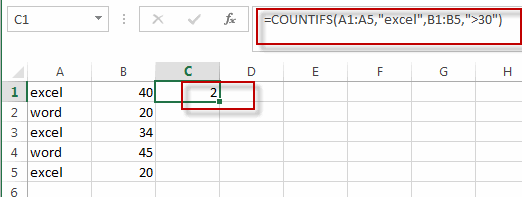### Related Functions

• Excel COUNTIF function
The Excel COUNTIF function will count the number of cells in a range that meet a given criteria. This function can be used to count the different kinds of cells with number, date, text values, blank, non-blanks, or containing specific characters.etc.= COUNTIF (range, criteria)…
• Excel SUMPRODUCT function
The Excel SUMPRODUCT function multiplies corresponding components in the given one or more arrays or ranges, and returns the sum of those products.The syntax of the SUMPRODUCT function is as below:= SUMPRODUCT (array1,[array2],…)…
• Excel SUM function
The Excel SUM function will adds all numbers in a range of cells and returns the sum of these values. You can add individual values, cell references or ranges in excel.The syntax of the SUM function is as below:= SUM(number1,[number2],…)…
• Excel COUNTIFS function
The Excel COUNTIFS function returns the count of cells in a range that meet one or more criteria. The syntax of the COUNTIFS function is as below:= COUNTIFS(criteria_range1, criteria1, [criteria_range2, criteria2]…)…

## Rank values in a column based a specific value in another column

This post will guide you how to rank values in a column based on a specific value in another column in excel. How do I rank values in a range by a specific value in another column in excel.

## Rank Values Based on a Specific Value

Assuming that you have a list of data contains two columns and the first column is product list and another is Sales number. You want to rank the sales number of a specified product name, and you are not able to use the RANK function, because this function is only used to rank a value in a range. So how to achieve it.

You can try to write a complex formula based on the IF function and the COUNTIFS function to achieve the result.

For example, you want to rank values in range B2:B6 based on a specified value “excel” in the range A2:A6, then you can use the following formula:

`=IF(A2="excel",COUNTIFS(A\$2:A\$6,"excel",B\$2:B\$6,">"&B2)+1,"")`

Type this formula in another blank cell and then press Enter key in your keyboard, and drag the AutoFill Handler over other cells to apply this formula.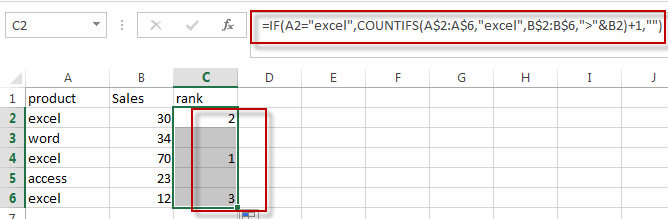You will see that the values in the range B2:B5 based on the “excel” in column A have been ranked.

### Related Functions

• Excel IF function
The Excel IF function perform a logical test to return one value if the condition is TRUE and return another value if the condition is FALSE. The IF function is a build-in function in Microsoft Excel and it is categorized as a Logical Function.The syntax of the IF function is as below:= IF (condition, [true_value], [false_value])….
• Excel Rank function
The Excel RANK function returns the rank of a given number in a supplied range of cells.  If there are duplicated values in a list of values, it will be set the same rank.The syntax of the RANK function is as below:= RANK (number,ref,[order])….
• Excel COUNTIFS function
The Excel COUNTIFS function returns the count of cells in a range that meet one or more criteria. The syntax of the COUNTIFS function is as below:= COUNTIFS(criteria_range1, criteria1, [criteria_range2, criteria2]…)…

## Highlight Duplicate Rows

This tutorial will teach you how to highlight duplicates rows using conditional formatting feature in Excel. In the previous post, we talked that how to change the color of rows based on a certain number or text or begin a specific character in a specified column. And this post will talk that how to highlight entire rows that are duplicates in excel 2016, 2013 or lower version. Or how to change the background color of duplicate rows.

## Highlight duplicate rows in only one column

If you data just have only one column in each rows, then you can following the below steps to highlight duplicate rows:

1# select the range of cells in that column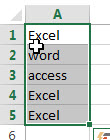2# on the HOME Tab, click Conditional Formatting command under Styles group, click Highlight Cells rules, and then select Duplicate Values.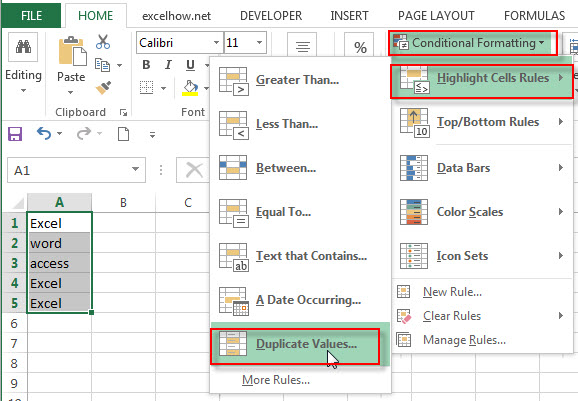3# select Duplicate and Light Red Fill with Dark Red Text from the Format cells that contains box in the Duplicate Values window. Click OK button.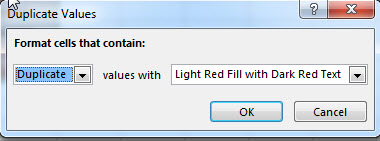4# you will see that all rows which are duplicates are highlighted.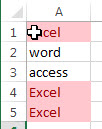## Highlight duplicate rows in multiple columns

Assuming that you want to highlight duplicate rows in a range of cells A2:C4 with conditional formatting, and you need to write a new formula to apply the conditions to find the duplicate rows.

Method 1:

You can create your own formula based on the COUNTIFS function to count duplicated values in each column of your range. So you can use the following formula with COUNTIFS function:

`=COUNTIFS(\$A\$2:\$A\$6,\$A2,\$B\$2:\$B\$6,\$B2,\$C\$2:\$C\$6,\$C2)>1`

Let’s see the below steps:

1# select the range of cells in your table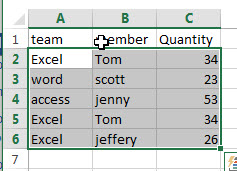2# on the HOME tab, click the Conditional Formatting command under Styles group. Then select New Rules… from the drop-down menu list.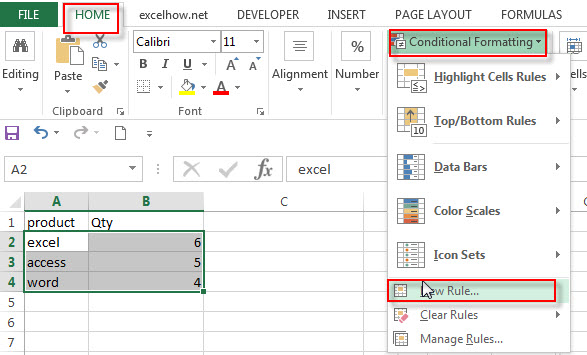3# the New Formatting Rule window will appear.

4# select the Use a formula to determine which cells to format option under Select a Rule Type: box, and then enter the above formula in the Format values where this formula is true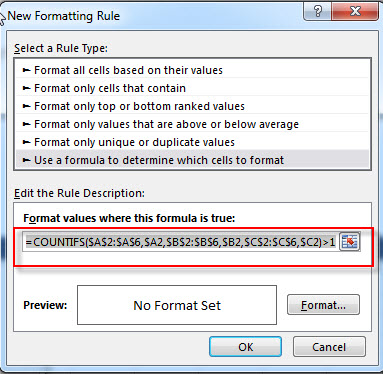5# click the Format… button, then the Format Cells window will appear.

6# in the “Format Cells” window, switch to the Fill tab, choose the background color, and then click OK button. you can also switch to other tabs to tweak the settings as you want.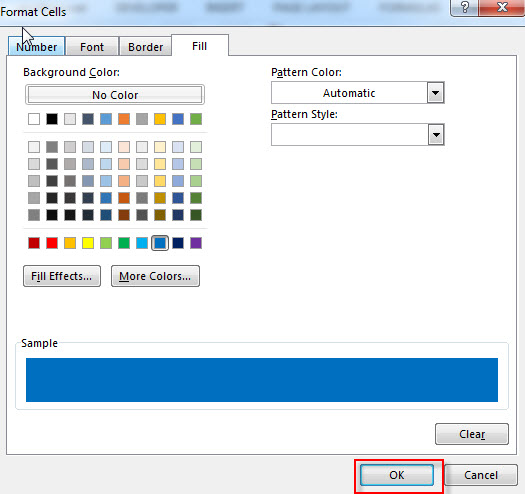7# back in the New Formatting Rule window, you can see a preview of your rows background color. Then click OK button.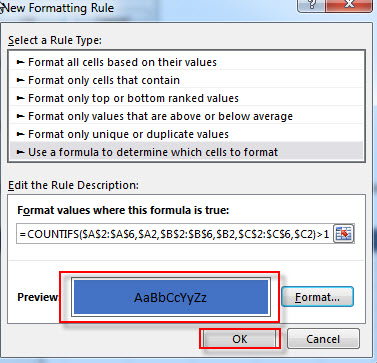8# let’s see the last result.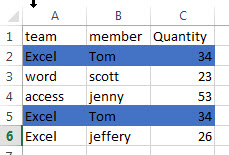If you just want to highlight duplicate rows except for the first occurrences, and you can use the following COUNTIFS formula as the conditional formatting rule.

`=COUNTIFS(\$A\$2:\$A2,\$A2,\$B\$2:\$B2,\$B2,\$C\$2:\$C2,\$C2)>1`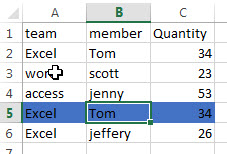Method 2:

You can use the CONCATENATE function or concatenate operator to join all values into one cell in each row, then you just need to check only one cell value in one column to find the duplicate values in the same column. At this time, you can use the COUNTIF function to create a formula, then apply it as the conditional formatting rule to find the duplicate rows. You can do the following steps:

1# create another column where you want to combine all values and call it Joined, such as column D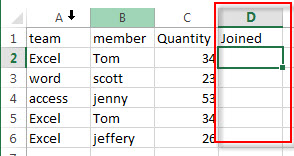2# enter the following formula into the cell D2 to combine columns in each row

`=CONCATENATE(A2,B2,C2)`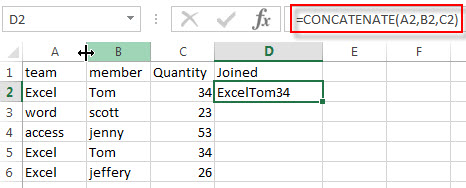3# drag AutoFill Handle down to the rest of cells in column D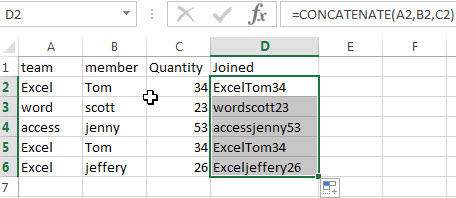4# select the range that you want to highlight duplicate rows including the column D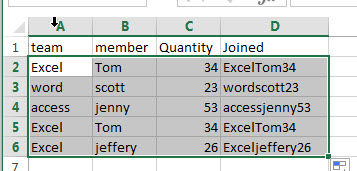5# on the HOME tab-> Styles -> Conditional Formatting, and then click New Rule….

6# select the Use a formula to determine which cells to format option under Select a Rule Type: box, and then enter the following formula in the Format values where this formula is true box.

`=COUNTIF(\$D\$2:\$D\$6,\$D2)>1`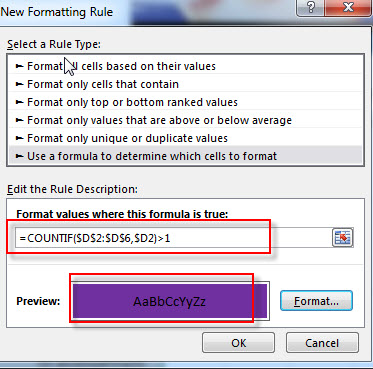7# click the Format… button, then switch to Fill tab, and choose one background color. Click OK.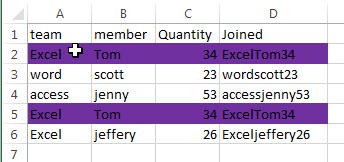8# you can  now delete the column D.

### Related Functions

• Excel Concat function
The excel CONCAT function combines 2 or more strings or ranges together.This is a new function in Excel 2016 and it replaces the CONCATENATE function.The syntax of the CONCAT function is as below:=CONCAT (text1,[text2],…)…
• Excel COUNTIFS function
The Excel COUNTIFS function returns the count of cells in a range that meet one or more criteria. The syntax of the COUNTIFS function is as below:= COUNTIFS(criteria_range1, criteria1, [criteria_range2, criteria2]…)…
• Excel COUNTIF function
The Excel COUNTIF function will count the number of cells in a range that meet a given criteria. This function can be used to count the different kinds of cells with number, date, text values, blank, non-blanks, or containing specific characters.etc.= COUNTIF (range, criteria)…

### Related Posts

• Highlight Rows
You will learn that how to change the color of the entire rows if the value of cells in a specified column meets your conditions, such as, if the value of cells is equal to or greater than a certain number or text values, then excel should be highlight entire rows or change a row color as you need.…
• Find Duplicate Rows
If you want to check the entire row that duplicated or not, if True, then returns “duplicates” value, otherwise, returns “no duplicates”. You can create a formula based on the IF function and the SUMPRODUCT function..…
• Highlight duplicate values
this post will teach you how to highlight duplicate values in the range of cells in excel. Normally, you may be need to identify duplicate values with a range of cells in Excel. And there is one of the fasted way that is using conditional formatting feature in Microsoft Excel……
• Combine Duplicate Rows and Sum the Values
This post will teach you how to combine duplicate rows and sum the corresponding values or calculate numbers in specific column in excel. And how to merge duplicate rows and then sum the values with VBA macro in Excel..…